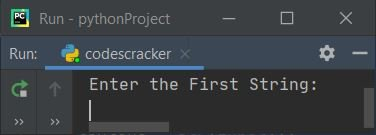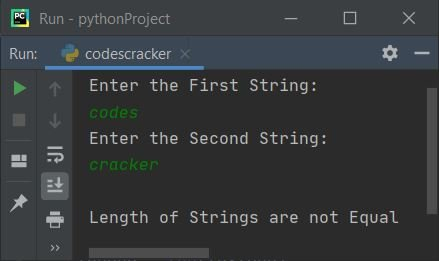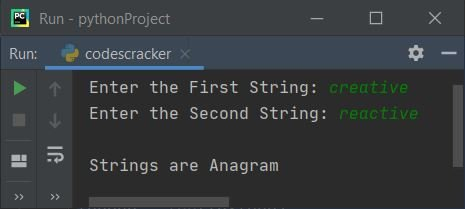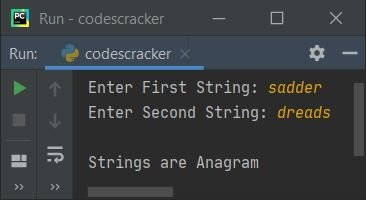# Python Program to Check If Two Strings Are Anagrams or Not

This article is created to cover some programs in Python that receive two strings from the user at run-time and check whether the two strings are anagrams or not. Here is a list of programs:

• Check if two strings are anagrams or not using the for loop without sorting the string.
• Check if two strings are anagrams or not using the sorted() function.
• Check if two strings are anagrams or not using a user-defined function.
• Check if two strings are anagrams or not using Class.

Before creating these programs, let's first understand anagrams.

### What are anagram strings?

Two strings can be called an anagram when:

• Both contain the same number of characters.
• The order of characters doesn't matter.
• One string can be rearranged to form the other one.

For example, abc and cba are two anagram strings. Similarly, creative and reactive are also anagrams. That is, characters from creative can be rearranged to form reactive, and vice versa.

## Check Two strings are anagrams using a for loop

This program is created using a for loop to check whether the two strings entered by the user are anagrams or not. The question is: write a Python program to check if an anagram exists or not without sorting the string. Here is its answer:

```print("Enter the First String:")
textOne = str(input())
print("Enter the Second String:")
textTwo = str(input())
found=0
notFound=0
lenOne = len(textOne)
lenTwo = len(textTwo)
if lenOne == lenTwo:
for i in range(lenOne):
found = 0
for j in range(lenOne):
if textOne[i] == textTwo[j]:
found = 1
break
if found==0:
notFound = 1
break
if notFound==1:
print("\nStrings are Not Anagram")
else:
print("\nStrings are Anagram")
else:
print("\nLength of Strings are not Equal")```

Here is its sample run:Now supply two strings input, say listen as the first string and silent as the second string, and press the ENTER key to check and print whether these two strings are anagrams or not, as shown in the snapshot given below:Here is another sample run with user input listen and silenx as the first and second strings:And here is the third sample run with user input codes and cracker as the first and second strings:Note: The str() method is used to convert any type of value to a string type. And the len() method returns the length of the string passed as its argument.

The dry run of the above program with user input (listen and silent) goes like this:

• Initial values: textOne = listen (entered by the user), textTwo = silent (entered by the user), found = 0, notFound = 0.
• Now, using the len() method, the length of the first and second strings get initialized to the lenOne and lenTwo variables, respectively. So lenOne = 6 and lenTwo = 6.
• Since the condition (of if) lenOne == lenTwo or 6 == 6 evaluates to true, program flow goes inside this if's body.
• The range() function returns a sequence of values, starting with 0 and incrementing by 1 each time, by default. Continues until lenOne-1, since lenOne is provided as its argument.
• Therefore, at first execution, i's value is 0, so i<lenOne or 0<6 evaluates to true, and therefore program flow goes inside this for loop's body.
• And 0 gets initialized to found. Now there is another for loop that gets executed.
• So j=0 and since 0 is less than lenOne's value, the condition evaluates to true, and therefore program flow goes inside this for loop's body.
• Inside this loop, I've compared the character at the current index of the first string to all the characters of the second string. That is, if the character of the first string matches any character of the second string, then 1 gets stored to the found variable, and using the break keyword, the remaining execution of this for loop gets skipped.
• And using the found==0 condition, we've checked whether program flow goes inside the previous if's body or not. That is, if the previous if's condition evaluates to true, then we've got to continue; otherwise, initialize 1 to notFound and, using the break keyword, skip the remaining execution of the outer for loop.
• In this way, I've compared in a character-by-character manner and checked whether two strings entered by the user are anagrams or not.
• Since each and every character of the first string is available in the second string, therefore program flow never goes inside the body of if whose condition is found==0. So that 1 never gets initialized to the notFound variable. And the condition notFound==1 doesn't evaluate to true, so else's statement gets executed.
• That prints Strings are Anagram.

#### Modified version of the previous program

This program uses the sorted() method to sort the string and compares strings in a character-by-character manner to check whether two strings are anagrams or not. The end= is used to skip inserting an automatic newline using print().

```print(end="Enter the First String: ")
textOne = str(input())
print(end="Enter the Second String: ")
textTwo = str(input())
lenOne = len(textOne)
lenTwo = len(textTwo)
notFound=0
if lenOne == lenTwo:
textOne = sorted(textOne)
textTwo = sorted(textTwo)
for i in range(lenOne):
if textOne[i] != textTwo[i]:
notFound=1
break
if notFound==0:
print("\nStrings are Anagram")
else:
print("\nStrings are Not Anagram")
else:
print("\nLength of Strings are not Equal")```

Here is its sample run with user input (creative and reactive) as two strings:## Check anagram strings using sorted()

This program uses the sorted() method to do the same job as the previous program did. The two strings entered by the user get compared directly using the == operator.

```print("Enter First String: ", end="")
textOne = str(input())
print("Enter Second String: ", end="")
textTwo = str(input())

lenOne = len(textOne)
lenTwo = len(textTwo)

if lenOne == lenTwo:
if sorted(textOne) == sorted(textTwo):
print("\nStrings are Anagram")
else:
print("\nStrings are Not Anagram")
else:
print("\nLength of Strings are not Equal")```## Check anagram strings using the function

This program is created using a user-defined function named CheckAnag(). The function receives two strings as its arguments and returns 1 if both strings are anagrams.

```def CheckAnag(sOne, sTwo):
if sorted(sOne) == sorted(sTwo):
return 1

print("Enter First String: ", end="")
textOne = str(input())
print("Enter Second String: ", end="")
textTwo = str(input())

rVal = CheckAnag(textOne, textTwo)
if rVal==1:
print("\nStrings are Anagram")
else:
print("\nStrings are Not Anagram")```

## Check anagram strings using Class

This is the last program created using classes, an object-oriented feature of Python. To access the member functions of a class, an object is required. Therefore, an object named obj is created of class CodesCracker to access its member function named CheckAnag() using the dot (.) operator.

```class CodesCracker:
def CheckAnag(self, sOne, sTwo):
if sorted(sOne) == sorted(sTwo):
return 1

print("Enter First String: ", end="")
textOne = str(input())
print("Enter Second String: ", end="")
textTwo = str(input())

obj = CodesCracker()
rVal = obj.CheckAnag(textOne, textTwo)

if rVal==1:
print("\nStrings are Anagram")
else:
print("\nStrings are Not Anagram")```

Python Online Test

« Previous Program Next Program »